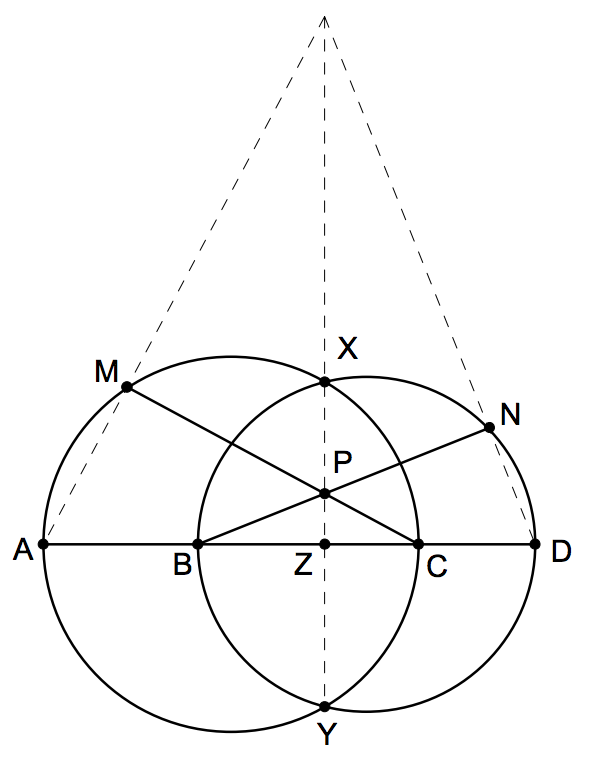TriangleCenter IMO
1995

Problem - 2455
Let $A$, $B$, $C$ and $D$ be four distinct points on a line, in that order. The circles with diameters $AC$ and $BD$ intersect at the points $X$ and $Y$. The line $XY$ meets $BC$ at the point $Z$. Let $P$ be a point on the line $XY$ different from $Z$. The line $CP$ intersects the circle with diameter $AC$ at the points $C$ and $M$, and the line $BP$ intersects the circle with diameter $BD$ at the points $B$ and $N$. Prove that the lines $AM$, $DN$ and $XY$ are concurrent